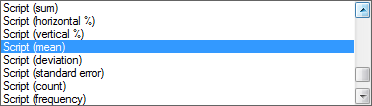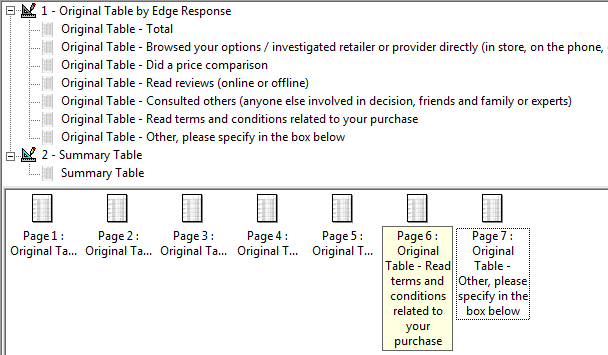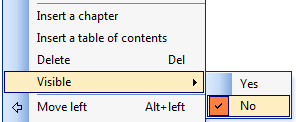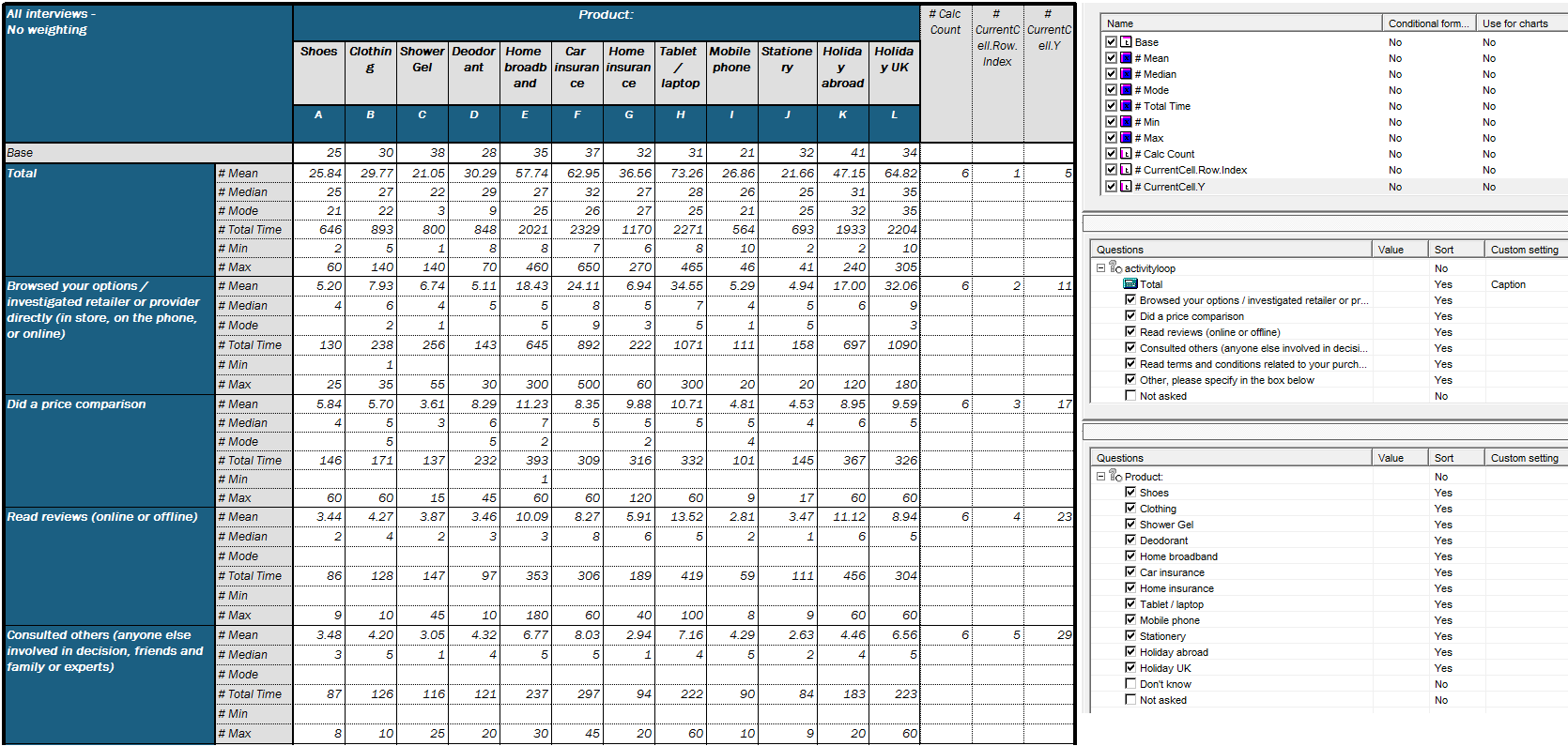Scroll

# Using table arithmetic to lookup other tables

Follow
 Summary This article shows the use of calculation arithmetic to look up data from one table layout (broken into separate tables by edge response) into another table layout (summary in single table). Applies to Askia Analyse. Written for Researchers, Developers, Data Processors. Keywords Table arithmetic, Forloops, Coordinates, data, Calculation arithmetic, Bespoke calculation, Look up, Edge, Break table.

The .qes file and portfolio containing the example discussed is attached: Table Arithmetic Examples 16.rar

There are some inbuilt calculations in Analyse such as Script (mean), Script (sum) etc. which would normally allow us to create a summary table. So why would we elect to use calculation arithmetic instead?

Well, in the example below we need to summarise min, max, mode & median values which are not supported in previously mentioned script (calculations).So in this case we can carry out a lookup using the GetTable method

GetTable ("Name as string", Table as optional number)   -   returns a table object

Broadly speaking, the method is to create a new tab definition which has the structure required and then do a lookup of the values from the original table(s) into this new table.

The original tables are broken by edge response to produce 7 tables:We will push data from these 7 separate tables into the new summary table. Then the original table(s) can then be made invisible so only the new table is exported when running your tabs (right-click on tab definition > Visible > No):To get the table structure you can set up like below (right) and we aim to get the results below (left).*The flat calculations (last three columns of the table) are not required for this summary table to work. They are simply shown to give a visualisation of the syntax references used.

Then in each of the calculations you will need to insert this lookup code:

`Dim rIndex = currentcell.row.indexGetTable("Original Table by Edge Response",rIndex).GetCell(CurrentCell.X,CurrentCell.Y  - (6 * (rIndex-1))).Value`

To explain a bit:

The tab definition Original Table by Edge Response produces 7 tables so we use the rIndex parameter in the  `GetTable("")`  syntax to select the correct one of the 7 tables depending on which row of the new table we are on.

The X co-ordinate of the values we are setting always remains the same between the table but the Y co-ordinate changes because the final table is much longer that the original. To manage this we add a bit of 'maths' to the Y- co-ordinate reference in the syntax:  `CurrentCell.Y  - (6 * (rIndex-1))).Value`

e.g. when we are on the category: 'Read reviews (online or offline)' our 'currentcell' Y co-ordinate values are 23 to 28 but in the original table they are still 5 to 10 so to transform this we add a negative (or upward) shift to the current cell Y co-ordinate.

the cell in old tab = current cell in new tab - (number of calculations * ( row questions's response index  -  1 ))

e.g.

5 = 23 - ( 6 * ( 4 - 1 ))
6 = 24 - ( 6 * ( 4 - 1 ))
...
10 = 28 - ( 6 * ( 4 - 1 ))  etc.

Now this syntax is fine but if you were to add or remove crossed calculations from the tables then you would need to change the '6' in the above syntax. Ideally, we want the syntax to be as dynamic as possible so we can use some other syntax to count the number of crossed calculations in your table - this is done by setting `nCalcY` in the below code:

`Dim y3Index = 0Dim nCalcY = 0For y3Index = CurrentTable.StartY+1 to CurrentTable.MaxYIf (CurrentTable.GetCell(CurrentTable.StartX,y3Index).Row.Index.ToInt() = 1) Then nCalcY = nCalcY + 1  EndifNext y3IndexDim rIndex = currentcell.row.indexGetTable("Original Table by Edge Response",currentcell.row.index).GetCell(CurrentCell.X,CurrentCell.Y  - (nCalcY * (rIndex-1))).Value`

Of course this is just one particular table layout. If you are interested, there are a couple of other examples in second attachment: Table Arithmetic Examples 16 (additional).rar

Was this article helpful?
0 out of 0 found this helpful
Have more questions? Submit a request